图像处理几何变换详解_mfc空间几何变换之图像平移、镜像、旋转、缩放详解 - CSDN
• 图像的旋转变换图像的位置变换，但旋转后图像的大小一般会改变。和平移变换一样，既可以把转出显示区域的图像截去，也可以扩大显示区域以显示完整的图像，如下图所示。 我们先讨论不裁剪转出部分，扩大显示区域...
旋转有一个绕着什么转的问题。通常的做法是以图像的中心为圆心旋转，将图像上的所有像素都旋转一个相同的角度。图像的旋转变换是图像的位置变换，但旋转后图像的大小一般会改变。和平移变换一样，既可以把转出显示区域的图像截去，也可以扩大显示区域以显示完整的图像，如下图所示。我们先讨论不裁剪转出部分，扩大显示区域的情况。在下图所示的平面坐标系中，A0逆时针旋转θ变成A1，r是该点到原点的距离，则旋转前：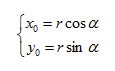旋转后A1的坐标为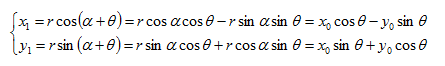写成矩阵的形式为：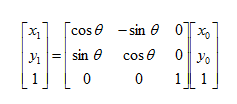其逆变换矩阵如下：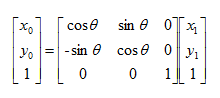上面公式是旋转变换的基本公式，坐标系是以图像的中心为原点，向右为x轴正方向，向上为y轴正方向。上述旋转是绕坐标原点进行的，如果是绕指定点（a,b）旋转，那么应该先将坐标系平移至改点，再旋转，然后平移至新的坐标原点。

下面推导坐标系平移的变换公式。坐标系Ⅰ是图像的坐标系，坐标系Ⅱ是旋转坐标系，坐标系Ⅱ的原点在坐标系中为（a,b），如下图所示。两种坐标系之间的转换为：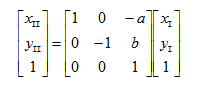逆变换为：有了上面的公式，就可以很方便的推导图像旋转变换的表达式。假设图像未旋转时候旋转中心的坐标是（a,b），旋转后中心点的坐标为（c,d）（在新的坐标系下，以旋转后图像的左上角为原点），则可以把变换分为3步：

第一步，将坐标系Ⅰ变成Ⅱ；

第二步，旋转θ（逆时针为正，顺时针为负）；

第三步，将坐标系Ⅱ变换回Ⅰ。这样就得到了总的变换矩阵。

设原图像某像素点的坐标为(x0，y0)，旋转后在目标图像的坐标为(x1，y1)，则旋转变换的矩阵表达式为：逆变换为：有了上面的转换公式，就可以很方便的编写出实现图像旋转的程序。首先需要计算出公式中需要的几个参数：a、b、c、d和旋转后图像的尺寸。已知原是图像的宽度为w0，高度为h0，以图像的中心为坐标原点。则原图像四个角的坐标分别是：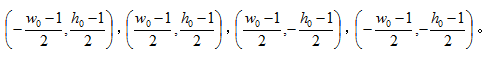按照旋转公式，旋转后这四个点的坐标分别是：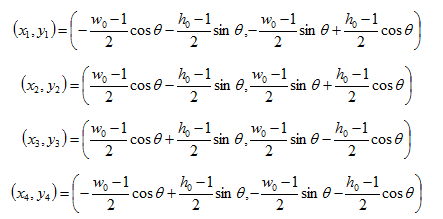则新图像的高度和宽度分别为：令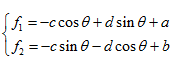图像旋转的主要代码如下：

void RotIamge(const Mat &srcImage, Mat &dstImage, double angle)
{
//弧度
double sita = angle * CV_PI / 180;
double a = (srcImage.cols - 1) / 2.0;
double b = (srcImage.rows - 1) / 2.0;

int srcRow = srcImage.rows;
int srcCol = srcImage.cols;

double x1 = -a * cos(sita) - b * sin(sita);
double y1 = -a * sin(sita) + b * cos(sita);

double x2 = a * cos(sita) - b * sin(sita);
double y2 = a * sin(sita) + b * cos(sita);

double x3 = a * cos(sita) + b * sin(sita);
double y3 = a * sin(sita) - b * cos(sita);

double x4 = -a * cos(sita) + b * sin(sita);
double y4 = -a * sin(sita) - b * cos(sita);

int w1 = cvRound(max(abs(x1 - x3), abs(x4 - x2)));
int h1 = cvRound(max(abs(y1 - y3), abs(y4 - y2)));
dstImage.create(h1, w1, srcImage.type());

double c = (w1 - 1) / 2.0;
double d = (h1 - 1) / 2.0;

double f1 = -c * cos(sita) + d * sin(sita) + a;
double f2 = -c * sin(sita) - d * sin(sita) + b;
int nRowNum = dstImage.rows;
int nColNum = dstImage.cols;
for (int i = 0; i < nRowNum; i++)
{

for (int j = 0; j < nColNum; j++)
{
int x = cvRound(j * cos(sita) - i * sin(sita) + f1);
int y = cvRound(j * sin(sita) + i * cos(sita) + f2);
if (x > 0 && x < srcCol && y > 0 && y < srcRow)
{
dstImage.at<Vec3b>(i, j) = srcImage.at<Vec3b>(y, x);
}
}
}
}

对于旋转以后图像大小不变的情况，旋转前后图像的中心点坐标都是（a,b），那么旋转的变换矩阵就是：逆变换为：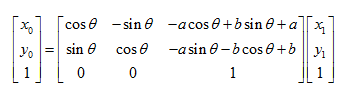公式中，主要代码如下：

void RotIamge2(const Mat &srcImage, Mat &dstImage, double angle)
{
//弧度
double sita = angle * CV_PI / 180;
double a = (srcImage.cols - 1) / 2.0 + 0.5;
double b = (srcImage.rows - 1) / 2.0 + 0.5;

int nRowNum = srcImage.rows;
int nColNum = srcImage.cols;
dstImage.create(nRowNum, nColNum, srcImage.type());

double f1 = -a * cos(sita) + b * sin(sita) + a;
double f2 = -a * sin(sita) - b * cos(sita) + b;

for (int i = 0; i < nRowNum; i++)
{
for (int j = 0; j < nColNum; j++)
{
int x = cvRound(j * cos(sita) - i * sin(sita) + f1);
int y = cvRound(j * sin(sita) + i * cos(sita) + f2);
if (x > 0 && x < nColNum && y > 0 && y < nRowNum)
{
dstImage.at<Vec3b>(i, j) = srcImage.at<Vec3b>(y, x);
}
}
}
}

要注意的是，由于有浮点运算，计算出来的坐标可能不是整数，需要采取取整处理，使用cvRound()函数寻找最接近的点，这样会带来一些误差，图像可能会出现锯齿，更好的方式是采用插值，后续将会具体介绍。

在opencv中可以使用getRotationMatrix2D()和warpAffine()实现任意角度的图像旋转。

Mat cv::getRotationMatrix2D  (Point2f center, double angle, double scale);

center：源图像中旋转的中心。
angle：旋转的角度值
scale：各向同性比例因子，指示图像是否缩小或放大
void cv::warpAffine(InputArray src, OutputArray dst, InputArray M, Size dsize, int flags = INTER_LINEAR, int borderMode BORDER_CONSTANT, const Scalar& borderValue = Scalar());

src：输入图像
dst：输出图像，其大小为dsize，并且与src类型相同。
M：2*3的变换矩阵，也就是getRotationMatrix2D返回的矩阵
dsize：输出图像的大小
flag：插值方法和可选标志WARP_INVERSE_MAP的组合，这意味着M是逆变换（dst→src）。
boardMode：像素外推方法； 当borderMode = BORDER_TRANSPARENT时，意味着目标图像中与源图像中的“离群值”相对应的像素未被该函数修改。
boardValue：在BORDER_CONSTANT情况下使用的值；默认情况下为0。
综合上述两个函数实现图像任意角度旋转的代码如下，这里借鉴了MATLAB中imrotate的处理方式：

void imrotate(InputArray src, OutputArray dst, double angle, int type)
{
CV_Assert( (angle >= -180) && (angle <= 180 ));
Mat srcMat = src.getMat();
if(srcMat.empty ())
{
src.copyTo (dst);
return;
}
int n = angle / 90;
double b = angle - int(angle / 90) * 90;
if(b == 0.0f)
{
switch(n)
{

case -2://旋转-180°
flip(srcMat, dst, -1);
break;
case -1: //旋转-90°
transpose (srcMat, dst);
flip(srcMat, dst, 1);
break;
case 0:
src.copyTo (dst);
break;
case 1://旋转90°
transpose (srcMat, dst);
flip(srcMat, dst, 0);
break;
case 2://旋转180°
flip(srcMat, dst, -1);
break;
default:
break;
}
}
else//旋转任意角度
{
Point2f center = Point2f(srcMat.cols / 2.0, srcMat.rows / 2.0);
Mat rotMat = getRotationMatrix2D(center, angle, 1.0);
Rect rc = RotatedRect(center, Size(srcMat.cols, srcMat.rows), angle).boundingRect();
rotMat.at<double>(0, 2) += rc.width / 2 - center.x;
rotMat.at<double>(1, 2) += rc.height / 2 - center.y;
warpAffine(srcMat, dst, rotMat, rc.size(), INTER_LINEAR, BORDER_CONSTANT, Scalar(255, 255, 255));
}
}

int main()
{
Mat dstImage;
namedWindow("源图像");
imshow("源图像", srcImage);
imrotate (srcImage, dstImage, 45, 0);
namedWindow("结果");
imshow("结果", dstImage);
waitKey(0);
}展开全文图像处理
• 该系列文章是讲解Python OpenCV图像处理知识，主要讲解图像入门、OpenCV基础用法。前面的第六篇文章讲解了图像缩放、图像旋转、图像翻转和图像平移的几何变换，本篇文章主要讲解图像仿射变换和图像透视变换，通过...
该系列文章是讲解Python OpenCV图像处理知识，前期主要讲解图像入门、OpenCV基础用法，中期讲解图像处理的各种算法，包括图像锐化算子、图像增强技术、图像分割等，后期结合深度学习研究图像识别、图像分类应用。希望文章对您有所帮助，如果有不足之处，还请海涵~
该系列在github所有源代码：https://github.com/eastmountyxz/ImageProcessing-Python
PS：请求帮忙点个Star，哈哈，第一次使用Github，以后会分享更多代码，一起加油。
同时推荐作者的C++图像系列知识：
[数字图像处理] 一.MFC详解显示BMP格式图片
[数字图像处理] 二.MFC单文档分割窗口显示图片
[数字图像处理] 三.MFC实现图像灰度、采样和量化功能详解
[数字图像处理] 四.MFC对话框绘制灰度直方图
[数字图像处理] 五.MFC图像点运算之灰度线性变化、灰度非线性变化、阈值化和均衡化处理详解
[数字图像处理] 六.MFC空间几何变换之图像平移、镜像、旋转、缩放详解
[数字图像处理] 七.MFC图像增强之图像普通平滑、高斯平滑、Laplacian、Sobel、Prewitt锐化详解
前文参考：
[Python图像处理] 一.图像处理基础知识及OpenCV入门函数
[Python图像处理] 二.OpenCV+Numpy库读取与修改像素
[Python图像处理] 三.获取图像属性、兴趣ROI区域及通道处理
[Python图像处理] 四.图像平滑之均值滤波、方框滤波、高斯滤波及中值滤波
[Python图像处理] 五.图像融合、加法运算及图像类型转换
[Python图像处理] 六.图像缩放、图像旋转、图像翻转与图像平移
[Python图像处理] 七.图像阈值化处理及算法对比
[Python图像处理] 八.图像腐蚀与图像膨胀
[Python图像处理] 九.形态学之图像开运算、闭运算、梯度运算
[Python图像处理] 十.形态学之图像顶帽运算和黑帽运算
[Python图像处理] 十一.灰度直方图概念及OpenCV绘制直方图
前面的第六篇文章讲解了图像缩放、图像旋转、图像翻转和图像平移的几何变换，本篇文章主要讲解图像仿射变换和图像透视变换，通过Python调用OpenCV函数实。基础性知识希望对您有所帮助。
1.图像仿射变换
2.图像透视变换
3.基于图像透视变换的图像校正
4.图像几何变换总结
PS：文章参考自己以前系列图像处理文章及OpenCV库函数，同时，本篇文章涉及到《计算机图形学》基础知识，请大家下来补充。
参考文献：
Python下opencv使用笔记（三）（图像的几何变换）
数字图像处理——图像的几何变换
图像校正-透视变换——t6_17

一.图像仿射变换
图像仿射变换又称为图像仿射映射，是指在几何中，一个向量空间进行一次线性变换并接上一个平移，变换为另一个向量空间。通常图像的旋转加上拉升就是图像仿射变换，仿射变换需要一个M矩阵实现，但是由于仿射变换比较复杂，很难找到这个M矩阵.
OpenCV提供了根据变换前后三个点的对应关系来自动求解M的函数——cv2.getAffineTransform(pos1,pos2)，其中pos1和pos2表示变换前后的对应位置关系，输出的结果为仿射矩阵M，接着使用函数cv2.warpAffine()实现图像仿射变换。图5-14是仿射变换的前后效果图。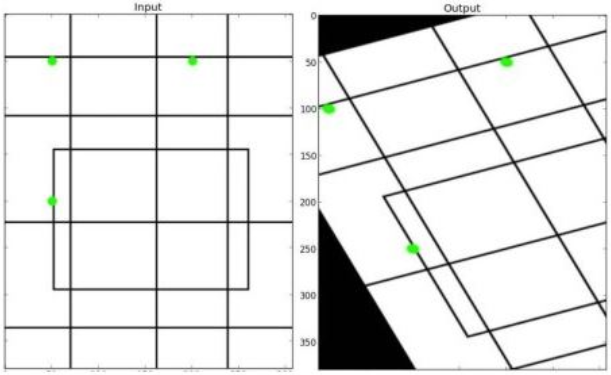图像仿射变换的函数原型如下：
M = cv2.getAffineTransform(pos1,pos2)

pos1表示变换前的位置
pos2表示变换后的位置

cv2.warpAffine(src, M, (cols, rows))

src表示原始图像
M表示仿射变换矩阵
(rows,cols)表示变换后的图像大小，rows表示行数，cols表示列数

实现代码如下所示：
#encoding:utf-8
import cv2
import numpy as np
import matplotlib.pyplot as plt

#读取图片

#获取图像大小
rows, cols = src.shape[:2]

#设置图像仿射变换矩阵
pos1 = np.float32([[50,50], [200,50], [50,200]])
pos2 = np.float32([[10,100], [200,50], [100,250]])
M = cv2.getAffineTransform(pos1, pos2)

#图像仿射变换
result = cv2.warpAffine(src, M, (cols, rows))

#显示图像
cv2.imshow("original", src)
cv2.imshow("result", result)

#等待显示
cv2.waitKey(0)
cv2.destroyAllWindows()

输出效果图如下所示：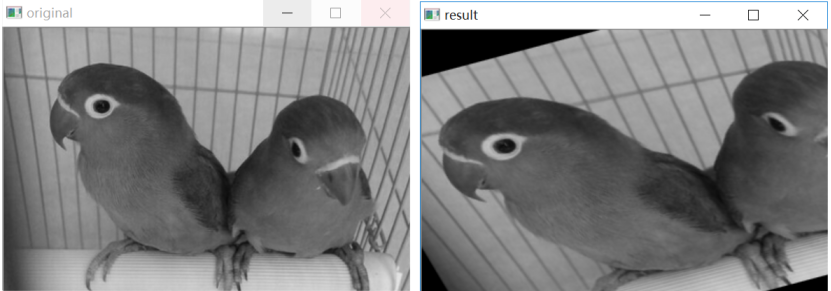二.图像透视变换
图像透视变换（Perspective Transformation）的本质是将图像投影到一个新的视平面，同理OpenCV通过函数cv2.getPerspectiveTransform(pos1,pos2)构造矩阵M，其中pos1和pos2分别表示变换前后的4个点对应位置。得到M后在通过函数cv2.warpPerspective(src,M,(cols,rows))进行透视变换。
图像透视变换的函数原型如下：
M = cv2.getPerspectiveTransform(pos1, pos2)

pos1表示透视变换前的4个点对应位置
pos2表示透视变换后的4个点对应位置

cv2.warpPerspective(src,M,(cols,rows))

src表示原始图像
M表示透视变换矩阵
(rows,cols)表示变换后的图像大小，rows表示行数，cols表示列数

代码如下：
#encoding:utf-8
import cv2
import numpy as np
import matplotlib.pyplot as plt

#读取图片

#获取图像大小
rows, cols = src.shape[:2]

#设置图像透视变换矩阵
pos1 = np.float32([[114, 82], [287, 156], [8, 322], [216, 333]])
pos2 = np.float32([[0, 0], [188, 0], [0, 262], [188, 262]])
M = cv2.getPerspectiveTransform(pos1, pos2)

#图像透视变换
result = cv2.warpPerspective(src, M, (190, 272))

#显示图像
cv2.imshow("original", src)
cv2.imshow("result", result)

#等待显示
cv2.waitKey(0)
cv2.destroyAllWindows()

输出结果如下图所示：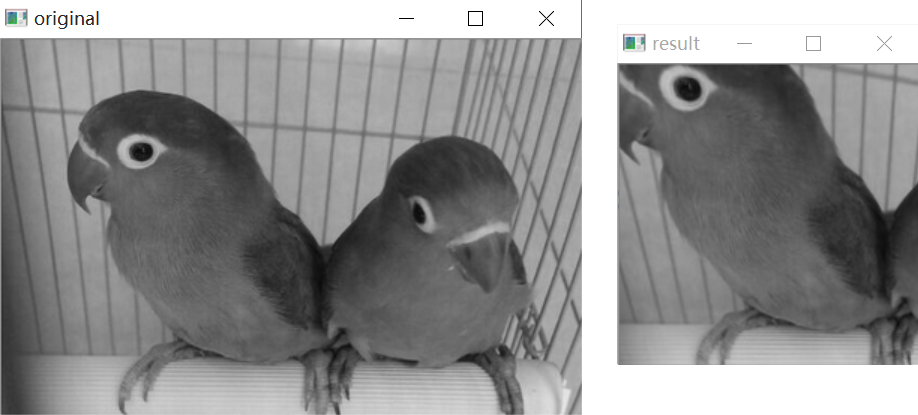三.基于图像透视变换的图像校正
下面参考 t6_17大神 的文章，通过图像透视变换实现图像校正功能。
假设现在存在一张A4纸图像，现在需要通过调用图像透视变换校正图像。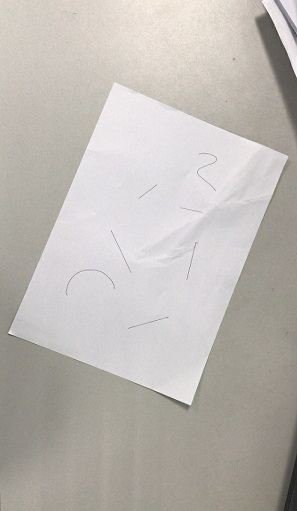代码如下所示：
#encoding:utf-8
import cv2
import numpy as np
import matplotlib.pyplot as plt

#读取图片

#获取图像大小
rows, cols = src.shape[:2]

#将源图像高斯模糊
img = cv2.GaussianBlur(src, (3,3), 0)
#进行灰度化处理
gray = cv2.cvtColor(img,cv2.COLOR_BGR2GRAY)

#边缘检测（检测出图像的边缘信息）
edges = cv2.Canny(gray,50,250,apertureSize = 3)
cv2.imwrite("canny.jpg", edges)

#通过霍夫变换得到A4纸边缘
lines = cv2.HoughLinesP(edges,1,np.pi/180,50,minLineLength=90,maxLineGap=10)

#下面输出的四个点分别为四个顶点
for x1,y1,x2,y2 in lines:
print(x1,y1),(x2,y2)
for x1,y1,x2,y2 in lines:
print(x1,y1),(x2,y2)

#绘制边缘
for x1,y1,x2,y2 in lines:
cv2.line(gray, (x1,y1), (x2,y2), (0,0,255), 1)

#根据四个顶点设置图像透视变换矩阵
pos1 = np.float32([[114, 82], [287, 156], [8, 322], [216, 333]])
pos2 = np.float32([[0, 0], [188, 0], [0, 262], [188, 262]])
M = cv2.getPerspectiveTransform(pos1, pos2)

#图像透视变换
result = cv2.warpPerspective(src, M, (190, 272))

#显示图像
cv2.imshow("original", src)
cv2.imshow("result", result)

#等待显示
cv2.waitKey(0)
cv2.destroyAllWindows()

运行结果如下图所示：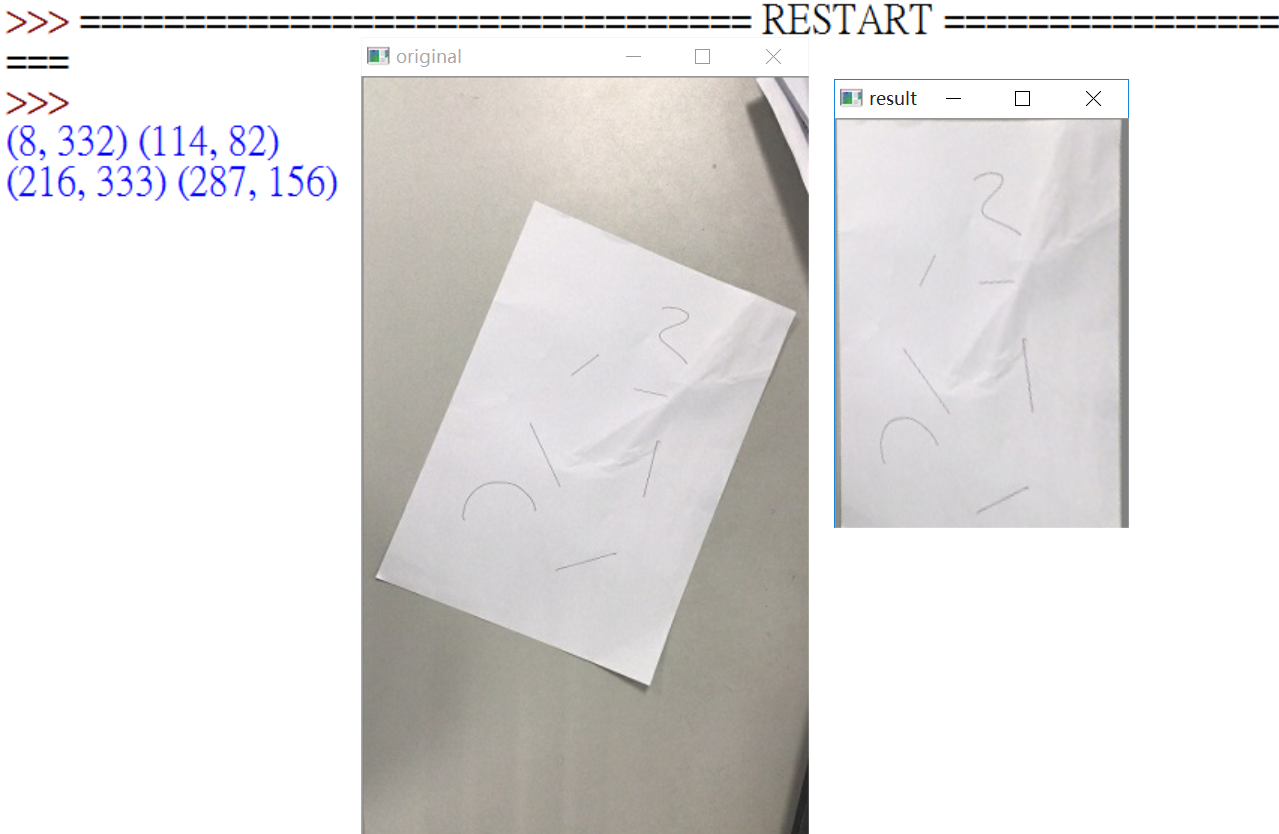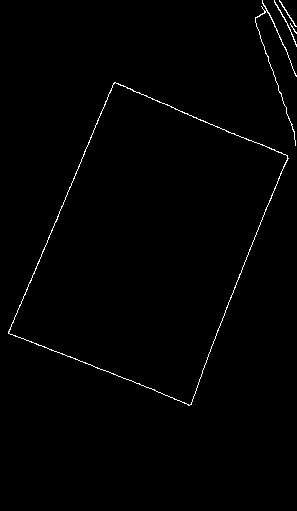四.图像几何变换总结
最后补充图像几何代码所有变换，希望读者能体会下相关的代码，并动手实践下。输出结果以女神为例：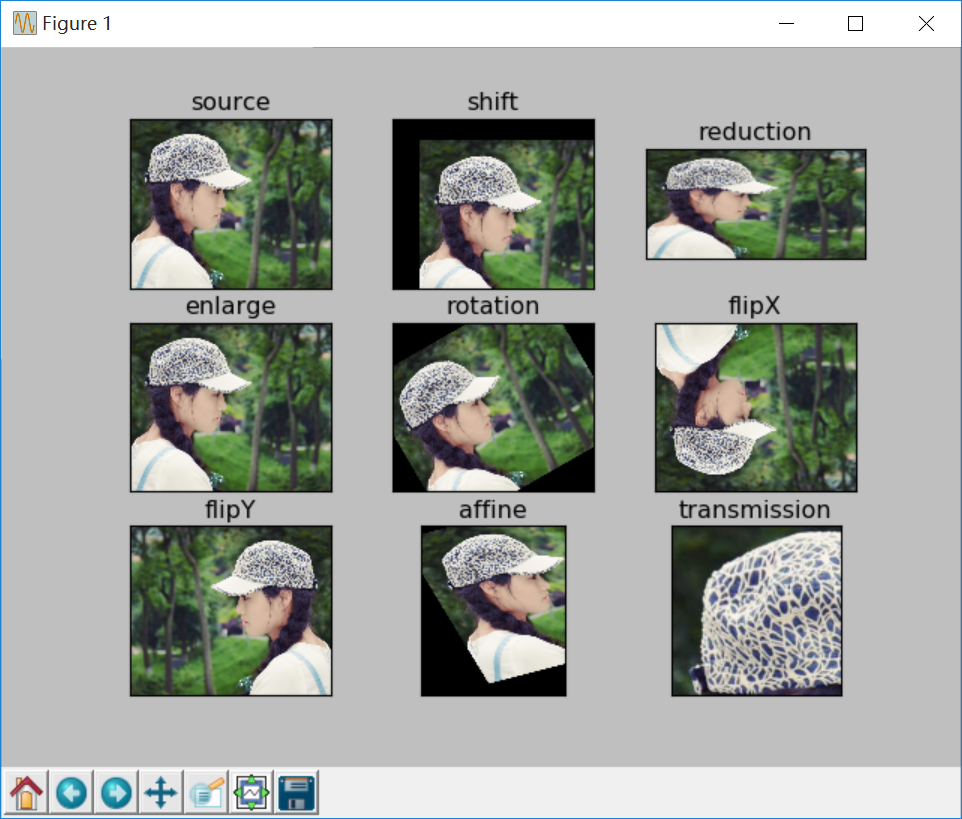完整代码如下：
#encoding:utf-8
import cv2
import numpy as np
import matplotlib.pyplot as plt

#读取图片
image = cv2.cvtColor(img,cv2.COLOR_BGR2RGB)

#图像平移矩阵
M = np.float32([[1, 0, 80], [0, 1, 30]])
rows, cols = image.shape[:2]
img1 = cv2.warpAffine(image, M, (cols, rows))

#图像缩小
img2 = cv2.resize(image, (200,100))

#图像放大
img3 = cv2.resize(image, None, fx=1.1, fy=1.1)

#绕图像的中心旋转
#源图像的高、宽 以及通道数
rows, cols, channel = image.shape
#函数参数：旋转中心 旋转度数 scale
M = cv2.getRotationMatrix2D((cols/2, rows/2), 30, 1)
#函数参数：原始图像 旋转参数 元素图像宽高
img4 = cv2.warpAffine(image, M, (cols, rows))

#图像翻转
img5 = cv2.flip(image, 0)   #参数=0以X轴为对称轴翻转
img6 = cv2.flip(image, 1)   #参数>0以Y轴为对称轴翻转

#图像的仿射
pts1 = np.float32([[50,50],[200,50],[50,200]])
pts2 = np.float32([[10,100],[200,50],[100,250]])
M = cv2.getAffineTransform(pts1,pts2)
img7 = cv2.warpAffine(image, M, (rows,cols))

#图像的透射
pts1 = np.float32([[56,65],[238,52],[28,237],[239,240]])
pts2 = np.float32([[0,0],[200,0],[0,200],[200,200]])
M = cv2.getPerspectiveTransform(pts1,pts2)
img8 = cv2.warpPerspective(image,M,(200,200))

#循环显示图形
titles = [ 'source', 'shift', 'reduction', 'enlarge', 'rotation', 'flipX', 'flipY', 'affine', 'transmission']
images = [image, img1, img2, img3, img4, img5, img6, img7, img8]
for i in xrange(9):
plt.subplot(3, 3, i+1), plt.imshow(images[i], 'gray')
plt.title(titles[i])
plt.xticks([]),plt.yticks([])
plt.show()


希望文章对大家有所帮助，如果有错误或不足之处，还请海涵。最近连续奔波考博，经历的事情太多，有喜有悲，需要改变自己好好对家人，也希望读者与我一起加油。
（By：Eastmount 2019-03-20 早上12点 https://blog.csdn.net/Eastmount/）


展开全文• 本文主要讲述基于VC++6.0 MFC图像处理的应用知识，主要结合自己大三所学课程《数字图像处理》及课件进行讲解，主要通过MFC单文档视图实现显示BMP图片空间几何变换，包括图像平移、图形旋转、图像反转倒置镜像和图像...
       本文主要讲述基于VC++6.0 MFC图像处理的应用知识，主要结合自己大三所学课程《数字图像处理》及课件进行讲解，主要通过MFC单文档视图实现显示BMP图片空间几何变换，包括图像平移、图形旋转、图像反转倒置镜像和图像缩放的知识。同时文章比较详细基础，没有采用GDI+获取矩阵，而是通过读取BMP图片信息头和矩阵像素实现变换，希望该篇文章对你有所帮助，尤其是初学者和学习图像处理的学生。
【数字图像处理】一.MFC详解显示BMP格式图片
【数字图像处理】二.MFC单文档分割窗口显示图片
【数字图像处理】三.MFC实现图像灰度、采样和量化功能详解
【数字图像处理】四.MFC对话框绘制灰度直方图
【数字图像处理】五.MFC图像点运算之灰度线性变化、灰度非线性变化、阈值化和均衡化处理详解
免费资源下载地址：
一. 图像平移

前一篇文章讲述了图像点运算(基于像素的图像变换)，这篇文章讲述的是图像几何变换：在不改变图像内容的情况下对图像像素进行空间几何变换的处理方式。        点运算对单幅图像做处理，不改变像素的空间位置；代数运算对多幅图像做处理，也不改变像素的空间位置；几何运算对单幅图像做处理，改变像素的空间位置，几何运算包括两个独立的算法：空间变换算法和灰度级插值算法。
空间变换操作包括简单空间变换、多项式卷绕和几何校正、控制栅格插值和图像卷绕，这里主要讲述简单的空间变换，如图像平移、镜像、缩放和旋转。主要是通过线性代数中的齐次坐标变换。
图像平移坐标变换如下：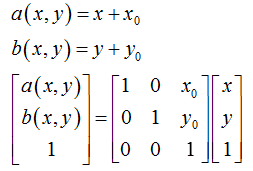运行效果如下图所示，其中BMP图片(0,0)像素点为左下角。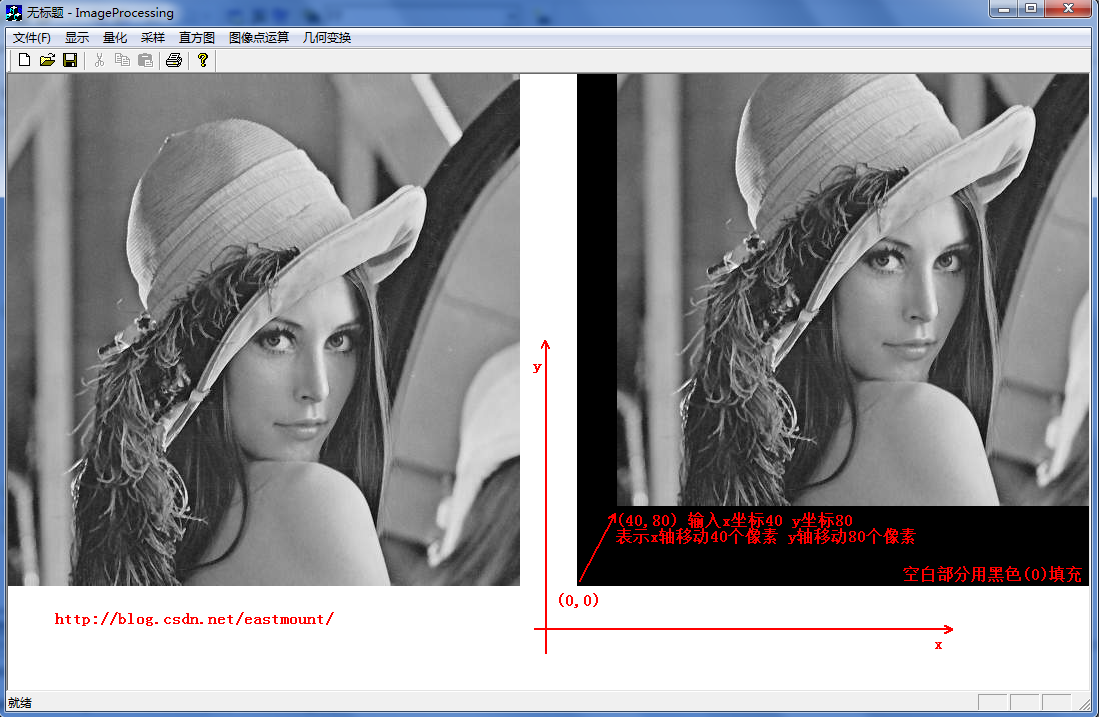其代码核心算法：
1.在对话框中输入平移坐标(x,y) m_xPY=x，m_yPY=y
2.定义Place=dlg.m_yPY*m_nWidth*3 表示当前m_yPY行需要填充为黑色
3.新建一个像素矩阵 ImageSize=new unsigned char[m_nImage]
4.循环整个像素矩阵处理
for(int i=0 ; i<m_nImage ; i++ ){
if(i<Place) {ImageSize[i]=black; continue;} //黑色填充底部 从小往上绘图                   else if(i>=Place && countWidth<dlg.m_xPY*3) {//黑色填充左部分                         ImageSize[i]=black; countWidth++;  continue;                   }                   else if(i>=Place && countWidth>=dlg.m_xPY*3) {//图像像素平移区域                        ImageSize[i]=m_pImage[m_pImagePlace];//原(0,0)像素赋值过去                        m_pImagePlace++; countWidth++;                        if(countWidth==m_nWidth*3) {
//一行填满 m_pImagePlace走到(0,1)                              number++; m_pImagePlace=number*m_nWidth*3;                        }                   }             }
5.写文件绘图fwrite(ImageSize,m_nImage,1,fpw)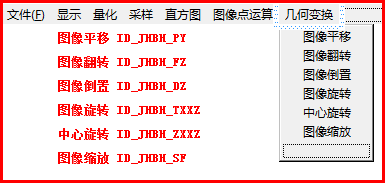第二步：设置平移对话框。将试图切换到ResourceView界面--选中Dialog,右键鼠标新建一个Dialog，并新建一个名为IDD_DIALOG_PY。编辑框(X)IDC_EDIT_PYX
和 (Y)IDC_EDIT_PYY，确定为默认按钮。设置成下图对话框：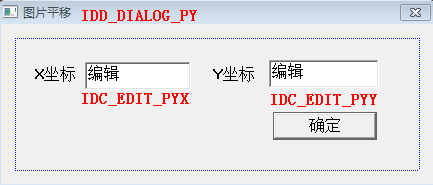第三步：在对话框资源模板空白区域双击鼠标—Create a new class创建一个新类--命名为CImagePYDlg。会自动生成它的.h和.cpp文件。打开类向导(Ctrl
W)，选择类名:CImagePYDlg添加成员变量如下图所示，同时在Message Maps中生成ID_JHBH_PY实现函数。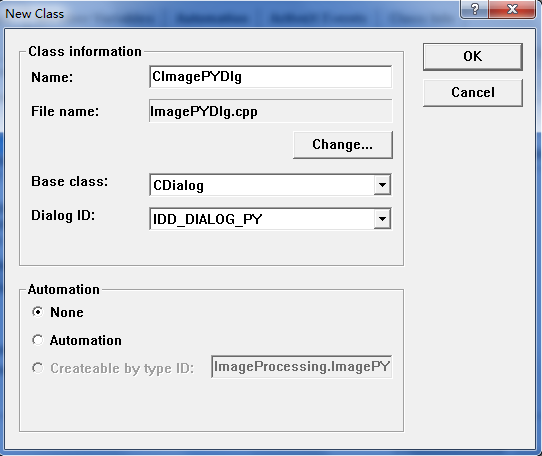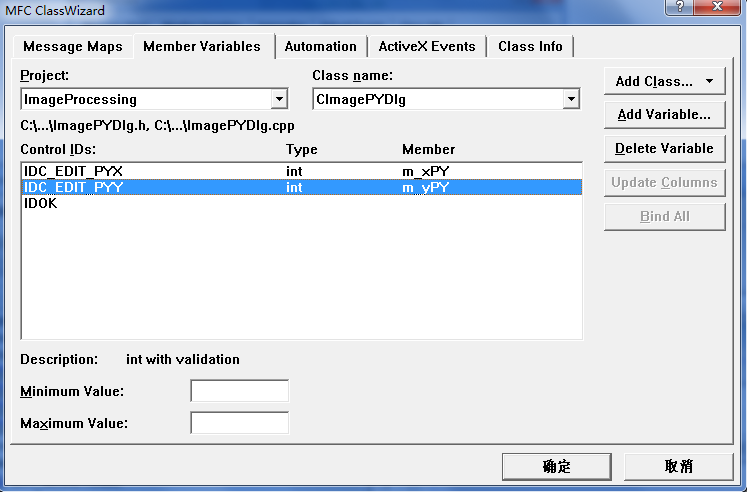第四步：在CImageProcessingView.cpp中添加头文件#include "ImagePYDlg.h"，并实现平移。/********************************************************/
/* 图像空间几何变换：图像平移 ID_JHBH_PY(几何变换-平移)
/* 使用平移对话框：CImagePYDlg dlg
/* 算法：f(x,y)=f(x+x0,y+y0)图像所有点平移，空的补黑'0'
/* 注意该图像平移方法只是从左上角(0,0)处开始平移
/* 其他方向原理相同 自己去实现
/********************************************************/

void CImageProcessingView::OnJhbhPy()
{
if(numPicture==0) {
AfxMessageBox("载入图片后才能空间平移!",MB_OK,0);
return;
}
//定义采样对话框也是用来空间变换平移的坐标
CImagePYDlg dlg;
if( dlg.DoModal()==IDOK ) //显示对话框
{
//采样坐标最初为图片的自身像素
if( dlg.m_xPY>m_nWidth || dlg.m_yPY>m_nHeight ) {
AfxMessageBox("图片平移不能为超过原图长宽!",MB_OK,0);
return;
}
AfxMessageBox("图片空间变换-平移!",MB_OK,0);

//打开临时的图片 读写文件
FILE *fpo = fopen(BmpName,"rb");
FILE *fpw = fopen(BmpNameLin,"wb+");

/************************************************************/
/* 图片空间变换-平移
/* 坐标(dlg.m_xPY,dlg.m_yPY)表示图像平移的坐标
/* 先用Plave计算出平移后的起始坐标，其他的坐标赋值为'0'黑色
/* 然后依次平移坐标，空的赋为黑色，否则填充
/************************************************************/

/******************************************************************/
/* 严重错误1：数组变量赋值相等
/* 在View.h中定义变量 BYTE *m_pImage 读入图片数据后的指针
/* 建立临时变量数组,让它平移变换 unsigned char *ImageSize
/* ImageSize=m_pImage(错误)
/* 会导致ImageSize赋值变换时m_pImage也产生了变换,所以输出全为黑色
/*     因为它俩指向了相同的数组地址
/* 解决方法：使用下面C++的new方法动态分配或for循环i=m_nImage赋值
/******************************************************************/

/*临时变量存储的像素与m_pImage相同，便于处理图像*/
unsigned char *ImageSize;
ImageSize=new unsigned char[m_nImage];  //new和delete有效的进行动态内存的分配和释放

int Place;                    //建立临时坐标 记录起始坐标(0,0)平移过来的位置
int m_pImagePlace;            //原始图像平移为(0,0) 图像把它平移到Place位置
unsigned char black;          //填充黑色='0'

/************************************************************/
/* for(int i=0 ; i<m_nHeight ; i++ )
/* for(int j=0 ; j<m_nWidth ; j++ )
/* 不能使用的上面的因为可能图像的最后一行没有完整的一行像素
/* 这样会出现exe报错,使用m_nImage读写所有像素比较正确
/************************************************************/

Place=dlg.m_yPY*m_nWidth*3;   //前m_yPY行都要填充为黑色
black=0;                       //颜色为黑色
m_pImagePlace=0;               //图像处事位置为(0,0),把该点像素平移过去
int countWidth=0;              //记录每行的像素个数,满行时变回0
int number=0;                  //数字记录使用的像素行数,平移时使用

for(int i=0 ; i<m_nImage ; i++ )
{
/*如果每行的像素填满时清为0*/
if(countWidth==m_nWidth*3) {
countWidth=0;
}

/*第一部分：到平移后像素位置前面的所有像素点赋值为黑色*/
if(i<Place) {
ImageSize[i]=black;     //赋值为黑色
continue;
}

/*第二部分：平移区域的左边部分赋值为黑色*/
else if(i>=Place && countWidth<dlg.m_xPY*3) { //RGB乘3
ImageSize[i]=black;     //赋值为黑色
countWidth++;
continue;
}

/****************************/
/* 各部分如图所示:
/* 000000000000000000000000
/* 000000000000000000000000
/* 0000000.................
/* 0000000.................
/* 0000000.................
/* 0000000.................
/* 点表示像素部分,0为黑色
/****************************/

/* 重点错误提示：由于bmp图像显示是从左下角开始存储(0,0)点所以输出图像为 */
/* bmp图像是从左下角到右上角排列的 */

/****************************/
/* 各部分如图所示:
/* 0000000.................
/* 0000000.................
/* 0000000.................
/* 0000000.................
/* 000000000000000000000000
/* 000000000000000000000000
/* 点表示像素部分,0为黑色
/****************************/

/*第三部分：图像像素平移区域*/
else if(i>=Place && countWidth>=dlg.m_xPY*3)
{
ImageSize[i]=m_pImage[m_pImagePlace];
m_pImagePlace++;
countWidth++;
if(countWidth==m_nWidth*3)
{
number++;
m_pImagePlace=number*m_nWidth*3;
}
}
}

fwrite(ImageSize,m_nImage,1,fpw);
fclose(fpo);
fclose(fpw);
numPicture = 2;
level=200;        //200表示几何变换
Invalidate();
}
}        同时在ShowBitmap中添加level标记重新绘制图片，代码如下：else        //图像几何变换
if(level=200)
{
}       运行时需要注意一点：BMP图像在处理过程中可能会出现一些斜线，而平移(40,60)位移量时可能出现如下。他是因为BMP格式有个非常重要的规定，要求每一扫描的字节数据必须能被4整除，也就是Dword对齐(长度4字节)，如果图像的一行字节数不能被4整除，就需要在每行末尾不起0达到标准。
例如一行像素为97字节，我们就需要补3个字节吗，数值可以是0，但是我们在BMP格式的信息头里说明了其宽度，所以补齐后对我们没有影响，所以后面补若干个字节的0即可直到被4整除。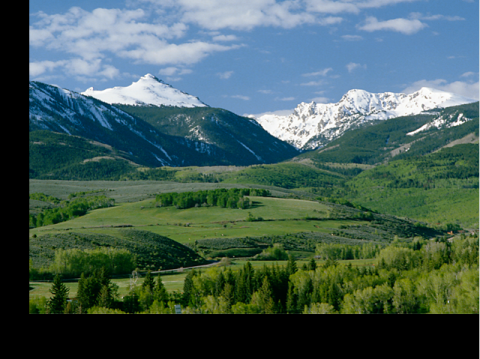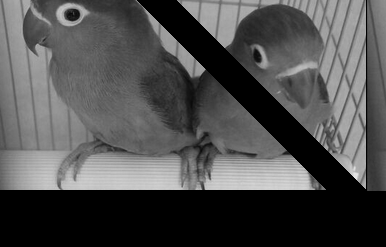通过后面的图像缩放后，我从学做了一遍这个补齐的缩放。代码如下，能够实现完美平移。nice啊~void CImageProcessingView::OnJhbhPy()
{
if(numPicture==0) {
AfxMessageBox("载入图片后才能空间平移!",MB_OK,0);
return;
}
//定义采样对话框也是用来空间变换平移的坐标
CImagePYDlg dlg;
if( dlg.DoModal()==IDOK ) //显示对话框
{
//采样坐标最初为图片的自身像素
if( dlg.m_xPY>m_nWidth || dlg.m_yPY>m_nHeight ) {
AfxMessageBox("图片平移不能为超过原图长宽!",MB_OK,0);
return;
}
AfxMessageBox("图片空间变换-平移!",MB_OK,0);

//打开临时的图片 读写文件
FILE *fpo = fopen(BmpName,"rb");
FILE *fpw = fopen(BmpNameLin,"wb+");

int num;            //记录每行多余的图像素数个数
int sfSize;         //补齐后的图像大小
//重点:图像的每行像素都必须是4的倍数：1*1的图像为 r g b 00H
if(m_nWidth*3%4!=0)
{
num=(4-m_nWidth*3%4);
sfSize=(m_nWidth*3+num)*m_nHeight; //每行多number个
}
else
{
num=0;
sfSize=m_nWidth*m_nHeight*3;
}
//注意:假如最后一行像素不足,我默认处理为完整的一行,不足补00H
//总之处理后的图像总是m*n且为4倍数,每行都完整存在

/*更改文件头信息 定义临时文件头结构变量*/
bfhsf=bfh;
bihsf=bih;
bfhsf.bfSize=sfSize+54;

CString str;
str.Format("补齐=%d",num);
AfxMessageBox(str);

/*临时变量存储的像素与sfSize相同 new和delete有效的进行动态内存的分配和释放*/
unsigned char *ImageSize;
ImageSize=new unsigned char[sfSize];

int Place;                    //建立临时坐标 记录起始坐标(0,0)平移过来的位置
int m_pImagePlace;            //原始图像平移为(0,0) 图像把它平移到Place位置
unsigned char black=0;        //填充黑色='0'
unsigned char other=0;        //补码00H='\0'

Place=dlg.m_yPY*(m_nWidth*3+num); //前m_yPY行都要填充为黑色
m_pImagePlace=0;                  //图像处事位置为(0,0),把该点像素平移过去
int countWidth=0;                 //记录每行的像素个数,满行时变回0
int number=0;                     //数字记录使用的像素行数,平移时使用

for(int i=0 ; i<sfSize ; i++ )
{
/*第一部分：到平移后像素位置前面的所有像素点赋值为黑色*/
if(i<Place)
{
ImageSize[i]=black;     //赋值为黑色
continue;
}

/*第二部分：平移区域的左边部分赋值为黑色*/
else if(i>=Place && countWidth<dlg.m_xPY*3)  //RGB乘3
{
ImageSize[i]=black;     //赋值为黑色
countWidth++;
continue;
}

/*第三部分：图像像素平移区域*/
else if(i>=Place && countWidth>=dlg.m_xPY*3)
{
ImageSize[i]=m_pImage[m_pImagePlace];
m_pImagePlace++;
countWidth++;
if(countWidth==m_nWidth*3)
{
if(num==0)
{
countWidth=0;
number++;
m_pImagePlace=number*m_nWidth*3;
}
else //num为补0
{
for(int j=0;j<num;j++)
{
i++;
ImageSize[i]=other;
}
countWidth=0;
number++;
m_pImagePlace=number*(m_nWidth*3+num); //重点：添加Num
}
}
}
}

fwrite(ImageSize,sfSize,1,fpw);
fclose(fpo);
fclose(fpw);
numPicture = 2;
level=200;        //200表示几何变换
Invalidate();
}
}        运行效果如下图所示，完美平移，其他算法遇到斜线问题类似补齐即可。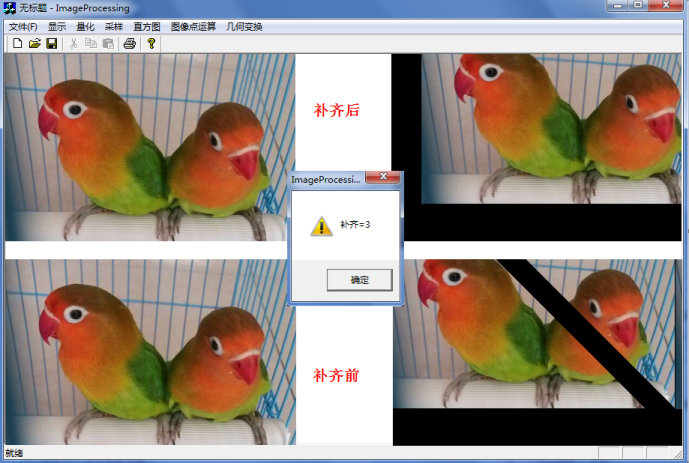二. 图像镜像
1.水平镜像翻转        其变换矩阵如下：                                 X=width-X0-1   (width为图像宽度)
Y=Y0        打开类向导，在CImageProcessingView中添加IDs为ID_JHBH_FZ，生成函数，代码如下：
/* 几何变换 图像翻转：自己对这个功能比较感兴趣,做个图像反转 */
void CImageProcessingView::OnJhbhFz()
{
if(numPicture==0) {
AfxMessageBox("载入图片后才能空间反转!",MB_OK,0);
return;
}
AfxMessageBox("图片空间变换-反转图像!",MB_OK,0);

//打开临时的图片
FILE *fpo = fopen(BmpName,"rb");
FILE *fpw = fopen(BmpNameLin,"wb+");

/*new和delete有效的进行动态内存的分配和释放*/
unsigned char *ImageSize;
ImageSize=new unsigned char[m_nImage];
int countWidth=0;        //记录每行的像素个数,满行时变回0
int Place;               //记录图像每行的位置,便于图像反转
int number=0;            //数字记录使用的像素行数
Place=m_nWidth*3-1;

//翻转矩阵: y=y0 x=width-x0-1
for(int i=0 ; i<m_nImage ; i++ )
{
if(countWidth==m_nWidth*3)
{
countWidth=0;
}
ImageSize[i]=m_pImage[Place]; //(0,0)赋值(0,width*3-1)像素
Place--;
countWidth++;
if(countWidth==m_nWidth*3)
{
number++;
Place=number*m_nWidth*3-1;
}
}

fwrite(ImageSize,m_nImage,1,fpw);
fclose(fpo);
fclose(fpw);
numPicture = 2;
level=200;
Invalidate();
}        运行效果如下图所示，其中还是存在一些小BUG，如前面的BMP图补0凑齐4整数倍宽度或颜色失帧。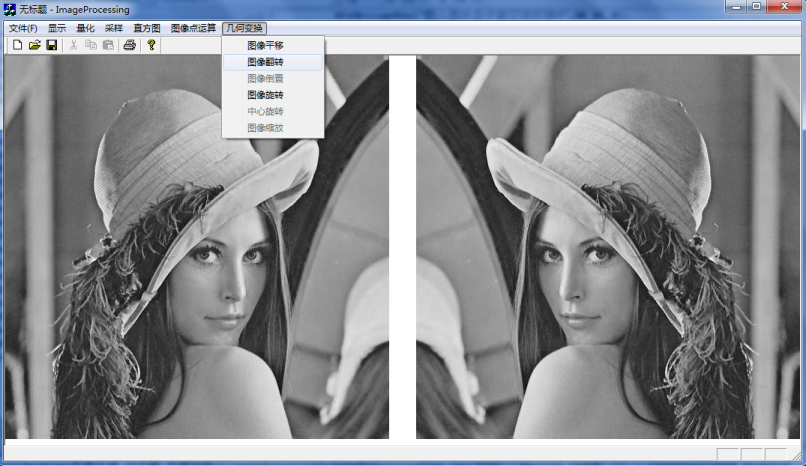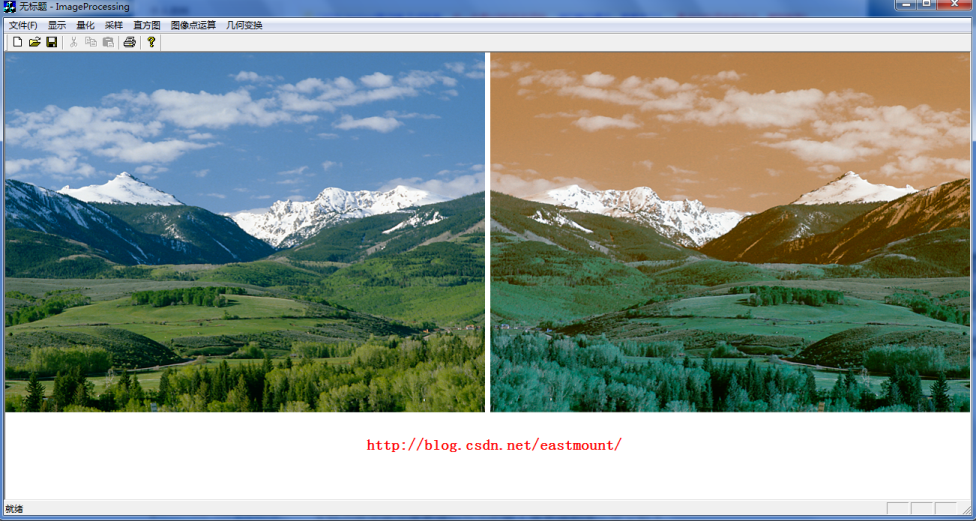2.垂直镜像倒转
其中变换矩阵如下：
X=X0
Y=height-Y0-1   (height为图像高度)        它相当于把原图的像素矩阵的最后一行像素值赋值给第一行，首先找到(0,0)对应的(height-1,0)像素值，然后依次赋值该行的像素数据；最后当前行赋值结束，依次下一行。重点是找到每行的第一个像素点即可。
代码中引用两个变量：Place=(m_nWidth*3)*(m_nHeight-1-1)即是(height-1,0)最后一行的第一个像素点；然后是循环中Place=(m_nWidth*3)*(m_nHeight-number-1)找到每行的第一个像素点。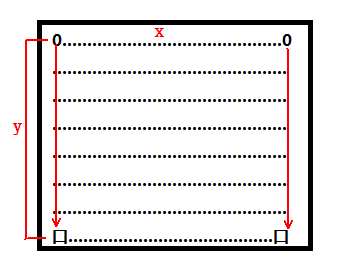同样通过类向导生成函数void CImageProcessingView::OnJhbhDz()，代码如下：/* 几何变换 图像倒转 */
void CImageProcessingView::OnJhbhDz()
{
if(numPicture==0) {
AfxMessageBox("载入图片后才能空间反转!",MB_OK,0);
return;
}
AfxMessageBox("图片空间变换-反转图像!",MB_OK,0);

//打开临时的图片
FILE *fpo = fopen(BmpName,"rb");
FILE *fpw = fopen(BmpNameLin,"wb+");

/*new和delete有效的进行动态内存的分配和释放*/
unsigned char *ImageSize;
ImageSize=new unsigned char[m_nImage];
int countWidth=0;                   //记录每行像素个数,满行时变回0
int Place;                          //每列位置
int number=0;                       //像素行数
Place=(m_nWidth*3)*(m_nHeight-1-1); //0行存储

//翻转矩阵: x=x0 y=height-y0-1
for(int i=0 ; i<m_nImage ; i++ )
{
ImageSize[i]=m_pImage[Place]; //(0,0)赋值(0,0)像素
Place++;
countWidth++;
if(countWidth==m_nWidth*3)
{
countWidth=0;
number++;
Place=(m_nWidth*3)*(m_nHeight-number-1);
}
}

fwrite(ImageSize,m_nImage,1,fpw);
fclose(fpo);
fclose(fpw);
numPicture = 2;
level=200;
Invalidate();
}        运行结果如下图所示，第二张图颜色没有失帧或变灰，这完全可以怀疑在翻转过程中RGB像素编程BGR后导致的结果，最终实现了翻转图像，但灰度存在一定；所以如果改为RBG顺序不变化即可原图颜色显示。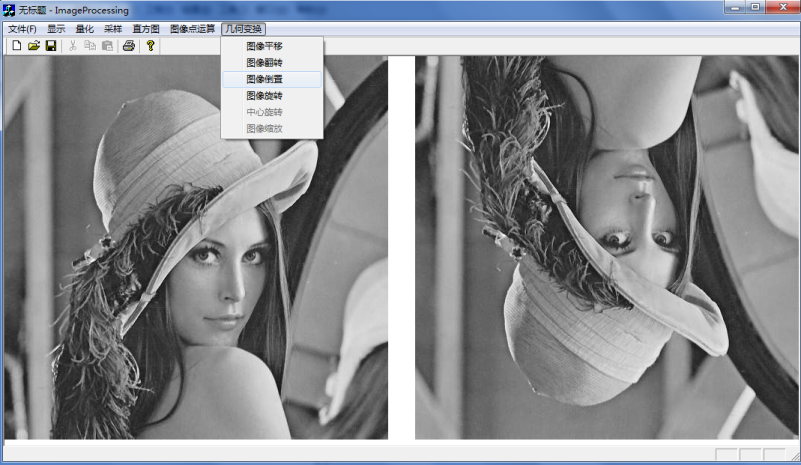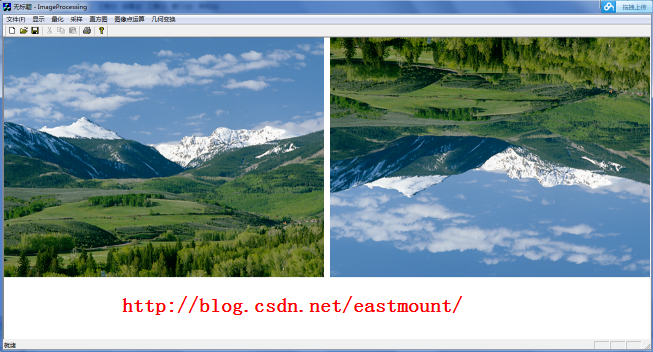三. 图像旋转
图像饶原点旋转顺时针theta角矩阵变换如下：注意BMP图像(0,0)左下角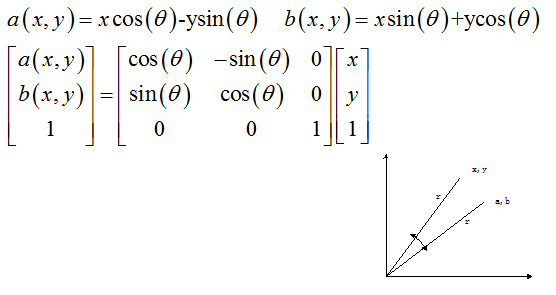写到这里真心觉得写底层的代码非常困难啊！尤其是以为像素转换二维像素，同时也觉得当时的自己算法部分还是很强大的，也感觉到如果采用GDI+操作像素矩阵Matrix或ColorMatrix是多么的方便，因为它定义好了X和Y向量，这就是为什么Android前面写的图像处理要容易得多。但是效率高~        好像利用GDI+旋转通过几句代码即可：
matrix.Rotate(15); //矩阵旋转15度
graph.SetTransform(&matrix);
graph.DrawImage(&image,points,3);
下面这部分代码是实现Android旋转的：参考我的博客//旋转图片
private void TurnPicture() {
Matrix matrix = new Matrix();
turnRotate=turnRotate+15;
//选择角度 饶(0,0)点选择 正数顺时针 负数逆时针 中心旋转
matrix.setRotate(turnRotate,bmp.getWidth()/2,bmp.getHeight()/2);
Bitmap createBmp = Bitmap.createBitmap(bmp.getWidth(), bmp.getHeight(), bmp.getConfig());
Canvas canvas = new Canvas(createBmp);
Paint paint = new Paint();
canvas.drawBitmap(bmp, matrix, paint);
imageCreate.setBackgroundColor(Color.RED);
imageCreate.setImageBitmap(createBmp);
textview2.setVisibility(View.VISIBLE);
}        实现效果如下图所示：言归正传，新建Dialog如下图所示，设置ID_DIALOG_XZ和变量：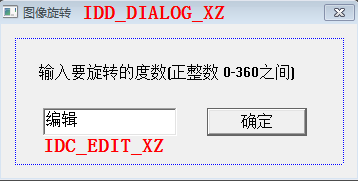再点击空白处创建CImageXZDlg类(旋转)，它会自动生成.h和.cpp文件。打开类向导生成CImageXZDlg类的成员变量m_xzds(旋转度数)，并设置其为int型(最大值360 最小值0)。
在类向导(Ctrl+W)选择类CImageProcessingView，为ID_JHBH_TXXZ(图像旋转)添加函数，同时添加头文件#include "ImageXZDlg.h"/**********************************************************/
/* 几何变换：图片旋转
/* 先添加对话框:IDD_JHBH_TXXZ(图像旋转),创建新类CImageXZDlg
/* 创建输入度数的:m_xzds Member variables 为int 0-360间
/**********************************************************/

void CImageProcessingView::OnJhbhTxxz()
{
if(numPicture==0) {
AfxMessageBox("载入图片后才能空间旋转!",MB_OK,0);
return;
}

//定义对话框并调用对话框
CImageXZDlg dlg;
if( dlg.DoModal()==IDOK ) //显示对话框
{
AfxMessageBox("图片空间变换-旋转图像!",MB_OK,0);
//读写文件
FILE *fpo = fopen(BmpName,"rb");
FILE *fpw = fopen(BmpNameLin,"wb+");

/*new和delete有效的进行动态内存的分配和释放*/
unsigned char *ImageSize;
ImageSize=new unsigned char[m_nImage];
int Place;          //记录图像每行的位置,便于图像旋转

/*定义PA=3.14时使用的方法是arcsin(1.0/2)*6即为π*/
double PA;
PA=asin(0.5)*6;

/*把输入的0-360的正整数度数转换为角度,30度=π/6*/
double degree;
degree=PA*dlg.m_xzds/180;   //调用dlg.m_xzds(旋转度数)

//对应的二维矩阵 注意图像矩阵从左下角开始处理 它最终要转换成一维存储
int X,Y;               //图像变换前通过一维矩阵转换为二维
int XPlace,YPlace;

//输出转换为的角度
CString str;
str.Format("转换后的角度=%f",degree);
AfxMessageBox(str);

//图像旋转处理
for(int i=0 ; i<m_nImage ; i++ )
{
//原图：一维矩阵转换为二维矩阵
X=(i/3)%m_nWidth;
Y=(i/3)/m_nWidth;
//注意错误：X=i/m_nHeight Y=i%m_nWidth; 只输出最后1/3

//图像旋转为：a(x,y)=x*cos-y*sin b(x,y)=x*sin+y*cos
XPlace=(int)(X*cos(degree)-Y*sin(degree));
YPlace=(int)(X*sin(degree)+Y*cos(degree));

//在转换为一维图想输出
if( (XPlace>=0 && XPlace<=m_nWidth) && (YPlace>=0 && YPlace<=m_nHeight) )
{
Place=YPlace*m_nWidth*3+XPlace*3;
//在图像范围内赋值为该像素
if(Place+2<m_nImage)
{
ImageSize[i]=m_pImage[Place];
i++;
ImageSize[i]=m_pImage[Place+1];
i++;
ImageSize[i]=m_pImage[Place+2];
}
//否则赋值为黑色
else
{
ImageSize[i]=0;
i++;
ImageSize[i]=0;
i++;
ImageSize[i]=0;
}
}
//否则赋值为黑色
else
{
ImageSize[i]=0;
i++;
ImageSize[i]=0;
i++;
ImageSize[i]=0;
}
}

fwrite(ImageSize,m_nImage,1,fpw);
fclose(fpo);
fclose(fpw);
numPicture = 2;
level=200;        //几何变换
Invalidate();
}
}        运行效果如下图所示，中心旋转太难了！找到中心那个位置就不太容易，我做不下去了，fuck~同时旋转过程中，由于是饶左下角(0,0)实现，故有的角度会到界面外显示全黑。下图分别旋转15度和355度。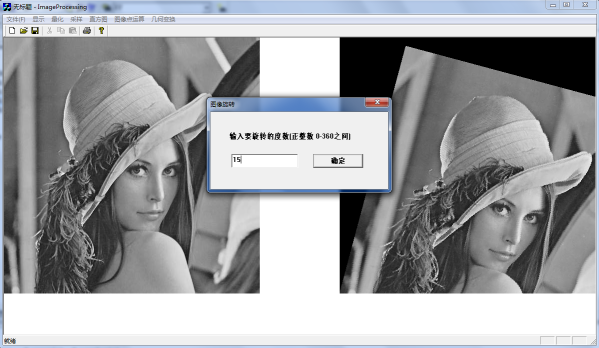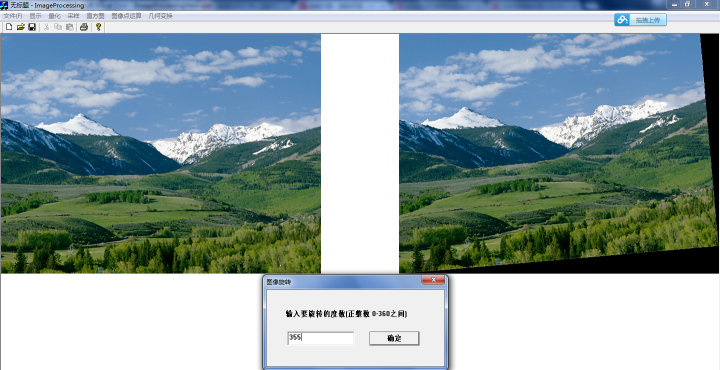四. 图像缩放
图像缩放主要有两种方法：
1.最近邻插值：向后映射时，输出图像的灰度等于离它所映射位置最近的输入图像的灰度值。其中向前映射和向后映射如下：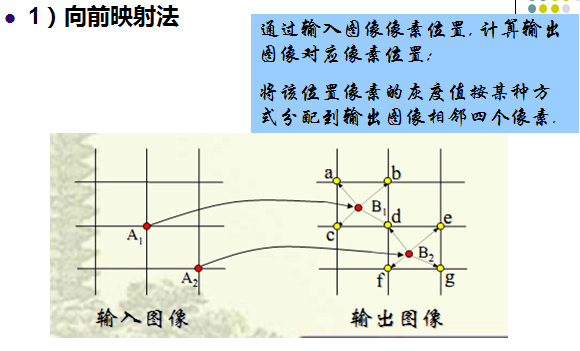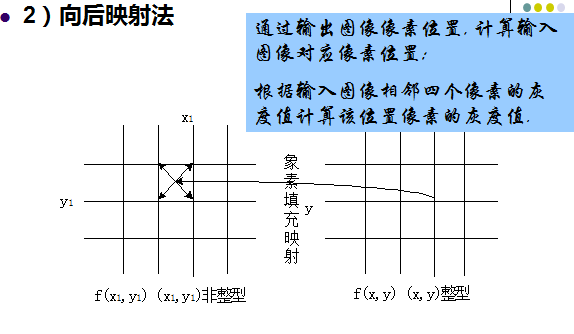对于向前映射每个输出图像的灰度要经过多次运算，对于向后映射，每个输出图像的灰度只经过一次运算。在实际应用中，更多的是采用向后映射法，其中根据四个相邻像素灰度值计算某个位置的像素灰度值即为灰度级插值。
2.双线性插值：四点确定一个平面函数，属于过约束问题。即单位正方形顶点已知，求正方形内任一点的f(x,y)值。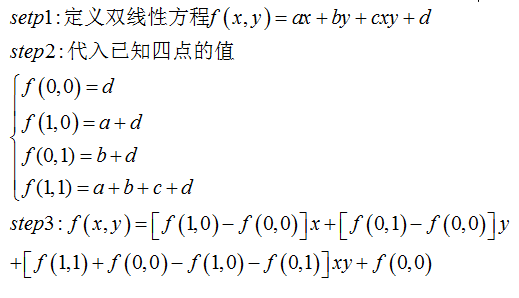换个通熟的说法，如下图所示。采用最近邻插值法就是P(x,y)像素值采用四舍五入等于离它最近的输入图像像素值。分别计算它到四个顶点之间的距离，但是这样会造成图像的马赛克、锯齿等现象。而采用双线性插值法，主要通过该坐标周围的四个像素值，按照比例混合计算器近似值。比例混合的依据是离哪个像素近，哪个像素的比例越大。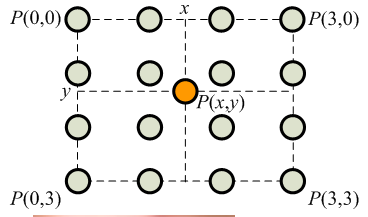下面是采用最近邻插值法的过程，注意BMP图缩放还需修改头文件信息。
第一步：在资源视图中添加“图像缩放”Dialog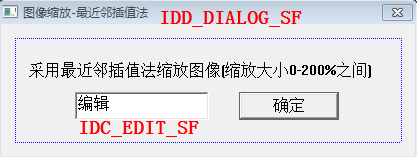第二步：点击空白处创建对话框的类CImageSFDlg，同时打开类向导为其添加成员变量m_sfbs(缩放倍数)，其为int型在0-200之间。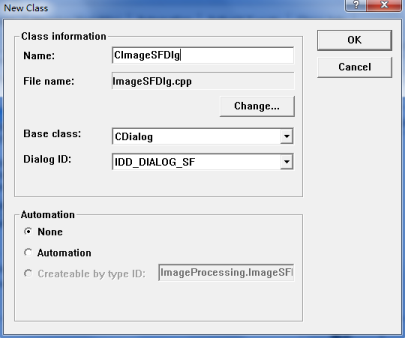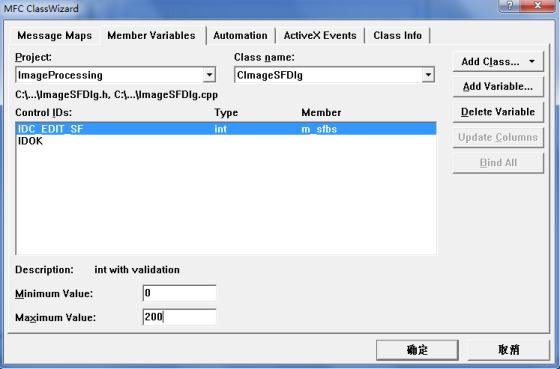第三步：打开类向导为其添加成员函数void CImageProcessingView::OnJhbhSf() 并实现缩放。同时添加头文件#include "ImageSFDlg.h"。/*******************************************************************/
/* ID_JHBH_SF: 几何运算-缩放-最近邻插值算法
/* 算法思想:输出图像的灰度等于离它所映射位置最近的输入图像的灰度值
/* 先计算出放大缩小后的长宽，根据它计算找原图中的点灰度，四舍五入
/*******************************************************************/

void CImageProcessingView::OnJhbhSf()
{
if(numPicture==0) {
AfxMessageBox("载入图片后才能几何缩放图像!",MB_OK,0);
return;
}

CImageSFDlg dlg;           //定义缩放对话框
if( dlg.DoModal()==IDOK )
{
//采样坐标最初为图片的自身像素  m_sfbs(缩放倍数)
if( dlg.m_sfbs==0 ) {
AfxMessageBox("输入图片缩放倍数不能为0!",MB_OK,0);
return;
}

FILE *fpo = fopen(BmpName,"rb");
FILE *fpw = fopen(BmpNameLin,"wb+");

/*先求缩放后的长宽*/
int sfWidth,sfHeight;                            //缩放后的长宽
int sfSize;                                      //缩放后的图像大小
sfWidth=(int)(m_nWidth*(dlg.m_sfbs*1.0)/100);    //24位图像RGB必须是3倍数 循环读取时为RGB
sfHeight=(int)(m_nHeight*(dlg.m_sfbs*1.0)/100);
int number;                                      //记录每行多余的图像素数个数

//重点:图像的每行像素都必须是4的倍数：1*1的图像为 r g b 00H
if(sfWidth*3%4!=0) {
number=(4-sfWidth*3%4);
sfSize=(sfWidth*3+(4-sfWidth*3%4))*sfHeight;
}
else {
number=0;
sfSize=sfWidth*sfHeight*3;
}
//注意:假如最后一行像素不足,我默认处理为完整的一行,不足补00H
//总之处理后的图像总是m*n且为4倍数,每行都完整存在

/*更改文件头信息 定义临时文件头结构变量*/
bfhsf=bfh;
bihsf=bih;

bfhsf.bfSize=sfSize+54;
bihsf.biWidth=sfWidth;
bihsf.biHeight=sfHeight;

//显示部分m_nDrawWidth<650显示原图,否则显示
flagSF=1;                         //图像缩放为1标识变量
m_nDrawWidthSF=sfWidth;
m_nDrawHeightSF=sfHeight;

unsigned char red,green,blue;
unsigned char other=0;                       //补码00H='\0'
int placeX;                                  //记录在原图中的第几行的位置
int placeY;									 //记录在原图中的位置(x,y)
int placeBH;                                 //记录变换后在变换图中的位置

/*new和delete有效的进行动态内存的分配和释放*/
unsigned char *ImageSize;
ImageSize=new unsigned char[sfSize];

/*读取文件像素信息 缩放注意:1.找最近灰度 2.四舍五入法(算法+0.5)*/
for(int i=0; i<sfHeight ; i++ )                  //行
{
placeX=(int)(i/(dlg.m_sfbs*1.0/100)+0.5)*bih.biWidth*3;
for(int j=0; j<sfWidth ; j++ )               //列
{
red=green=blue=0;
//放大倍数为(dlg.m_sfbs*1.0/100)
placeY=placeX+(int)(j/(dlg.m_sfbs*1.0/100)+0.5)*3;
//重点是:number*i补充00H,如果是numer图像会被切成2块
placeBH=(i*sfWidth*3+number*i)+j*3;
if(placeY+2<m_nImage)
{
ImageSize[placeBH]=m_pImage[placeY];
ImageSize[placeBH+1]=m_pImage[placeY+1];
ImageSize[placeBH+2]=m_pImage[placeY+2];
}
else
{
ImageSize[placeBH]=0;
ImageSize[placeBH+1]=0;
ImageSize[placeBH+2]=0;
}
}
}

fwrite(ImageSize,sfSize,1,fpw);
fclose(fpo);
fclose(fpw);
numPicture = 2;
level=200;
Invalidate();
}
}
        第四步：因为图像缩放修改BMP图片头信息，所以需要修改ShowBitmap中的显示第二张图片时的部分代码。如下所示：添加变量flagSF、m_nDrawWidthSF和m_nDrawHeightSF。/*定义显示图像缩放时的长宽与标记*/
int flagSF=0;          //图像几何变换缩放变换
int m_nDrawWidthSF=0;  //图像显示宽度缩放后
int	m_nDrawHeightSF=0; //图像显示高度缩放后

//****************显示BMP格式图片****************//
void CImageProcessingView::ShowBitmap(CDC *pDC, CString BmpName)
{
......
else        //图像几何变换
if(level=200)
{
}

if( m_bitmap.m_hObject ) {
m_bitmap.Detach();            //m_bitmap为创建的位图对象
}
m_bitmap.Attach(m_hBitmapChange);
//定义并创建一个内存设备环境
CDC dcBmp;
if( !dcBmp.CreateCompatibleDC(pDC) )   //创建兼容性的DC
return;
BITMAP m_bmp;                          //临时bmp图片变量
m_bitmap.GetBitmap(&m_bmp);            //将图片载入位图中
CBitmap *pbmpOld = NULL;
dcBmp.SelectObject(&m_bitmap);         //将位图选入临时内存设备环境

//图片显示调用函数StretchBlt
if(flagSF==1)
{
CString str;
str.Format("缩放长=%d 宽%d 原图长=%d 宽=%d",m_nDrawWidthSF,
m_nDrawHeightSF,m_nWidth,m_nHeight);
AfxMessageBox(str);
flagSF=0;
//m_nDrawWidthSF缩放此存见函数最近邻插值法中赋值
if(m_nDrawWidthSF<650 && m_nDrawHeightSF<650)
pDC->StretchBlt(m_nWindowWidth-m_nDrawWidthSF,0,
m_nDrawWidthSF,m_nDrawHeightSF,&dcBmp,0,0,m_bmp.bmWidth,m_bmp.bmHeight,SRCCOPY);
else
pDC->StretchBlt(m_nWindowWidth-640,0,640,640,&dcBmp,0,0,
m_bmp.bmWidth,m_bmp.bmHeight,SRCCOPY);  //显示大小为640*640
}
else {
//如果图片太大显示大小为固定640*640 否则显示原图大小
if(m_nDrawWidth<650 && m_nDrawHeight<650)
pDC->StretchBlt(m_nWindowWidth-m_nDrawWidth,0,
m_nDrawWidth,m_nDrawHeight,&dcBmp,0,0,m_bmp.bmWidth,m_bmp.bmHeight,SRCCOPY);
else
pDC->StretchBlt(m_nWindowWidth-640,0,640,640,&dcBmp,0,0,
m_bmp.bmWidth,m_bmp.bmHeight,SRCCOPY);
}
//恢复临时DC的位图
dcBmp.SelectObject(pbmpOld);
}        运行效果如下图所示，采用最近邻插值法缩放大了会出现失帧。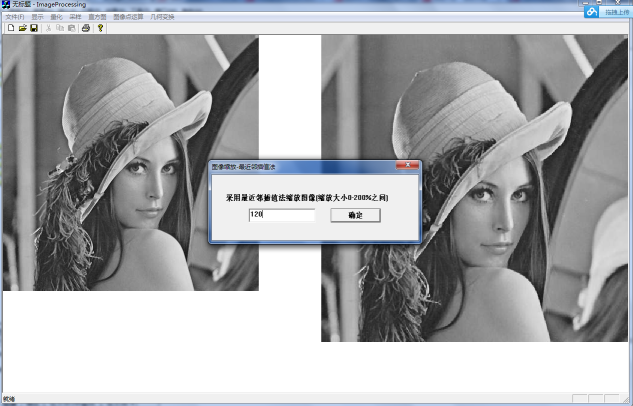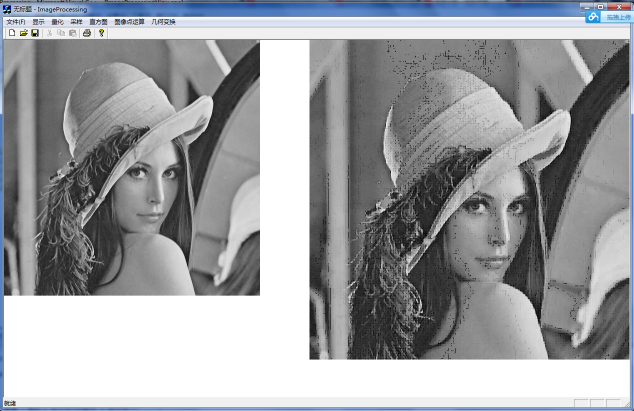但是同时当图片缩小是总是报错，图片缩放确实有点难，因为像素需要补齐4整数倍，同时需要修改消息头，同时像素矩阵的变换都非常复杂。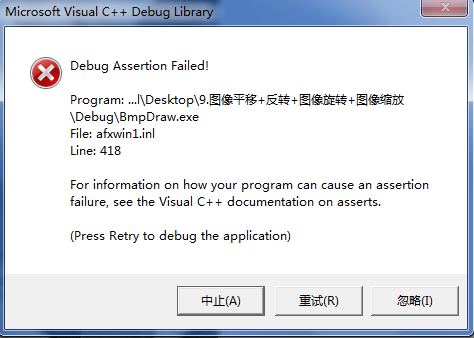最后还是希望文章对你有所帮助，如果文章有不足或错误之处，请海涵。自己给自己点个赞，挺不容易的，但还会继续写完~

（By:Eastmount 2015-06-04 下午5点   http://blog.csdn.net/eastmount/）


展开全文图像处理 几何变换
• # Task02 几何变换 ...在传统CV领域，由于某些拍摄角度的问题，我们需要对图像进行矫正处理，而几何变换正是这个处理过程的基础，因此了解和学习几何变换也是有必要的。 这次我们带着几个问题进行，以旋转为...
# Task02 几何变换
2.1 简介
该部分将对基本的几何变换进行学习，几何变换的原理大多都是相似，只是变换矩阵不同，因此，我们以最常用的平移和旋转为例进行学习。在深度学习领域，我们常用平移、旋转、镜像等操作进行数据增广；在传统CV领域，由于某些拍摄角度的问题，我们需要对图像进行矫正处理，而几何变换正是这个处理过程的基础，因此了解和学习几何变换也是有必要的。
这次我们带着几个问题进行，以旋转为例：

1：变换的形式（公式）是什么？

2：旋转中心是什么？毕竟以不同位置为旋转中心得到的结果是不一样的。

3：采用前向映射还是反向映射？(反向映射更为有效)

4：采用反向映射后，采用何种插值算法？最常用的的是双线性插值，OpenCV也是默认如此。

2.2 学习目标

了解几何变换的概念与应用

理解平移、旋转的原理

掌握在OpenCV框架下实现平移、旋转操作

2.3 内容介绍
1、平移、旋转的原理
2、OpenCV代码实践
3、动手实践并打卡（读者完成）
2.4 算法理论介绍
变换形式
先看第一个问题，变换的形式。与OpencV不同的是这里采取冈萨雷斯的《数字图像处理_第三版》的变换矩阵方式，关于OpenCV的策略可以看它的官方文档。根据冈萨雷斯书中的描述，仿射变换的一般形式如下：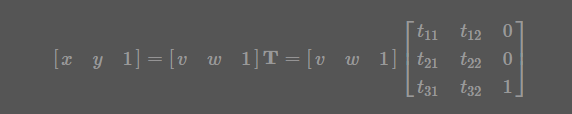式中的T就是变换矩阵，其中 (v,w)为原坐标，(x,y) 为变换后的坐标，不同的变换对应不同的矩阵，这里也贴出来吧，一些常见的变换矩阵及作用如下表：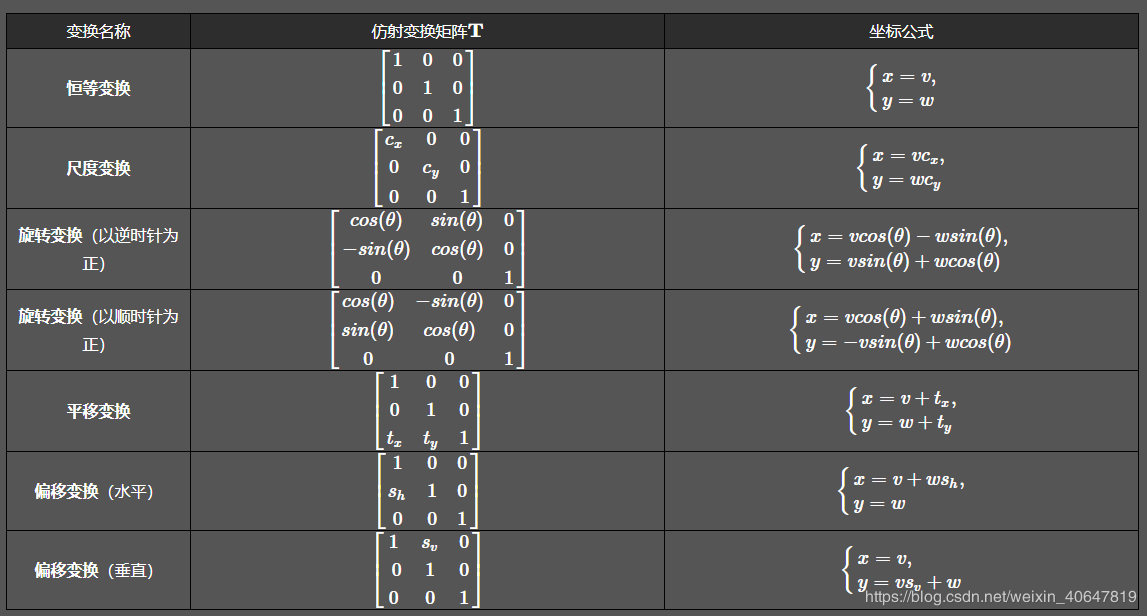也就是说，我们根据自己的目的选择不同变换矩阵就可以了。
坐标系变换
再看第二个问题，变换中心，对于缩放、平移可以以图像坐标原点（图像左上角为原点）为中心变换，这不用坐标系变换，直接按照一般形式计算即可。而对于旋转和偏移，一般是以图像中心为原点，那么这就涉及坐标系转换了。
我们都知道，图像坐标的原点在图像左上角，水平向右为 X 轴，垂直向下为 Y 轴。数学课本中常见的坐标系是以图像中心为原点，水平向右为 X 轴，垂直向上为 Y 轴，称为笛卡尔坐标系。看下图: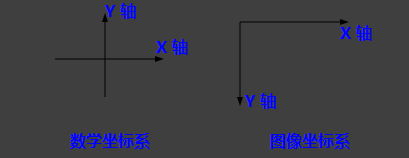因此，对于旋转和偏移，就需要3步（3次变换）：

将输入原图图像坐标转换为笛卡尔坐标系；
进行旋转计算。旋转矩阵前面已经给出了；
将旋转后的图像的笛卡尔坐标转回图像坐标。

图像坐标系与笛卡尔坐标系转换关系：
先看下图：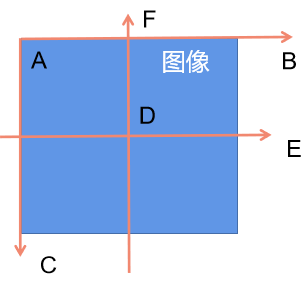在图像中我们的坐标系通常是AB和AC方向的,原点为A，而笛卡尔直角坐标系是DE和DF方向的，原点为D。
令图像表示为M×N的矩阵，对于点A而言，两坐标系中的坐标分别是(0，0)和(-N/2,M/2)，则图像某像素点(x’,y’)转换为笛卡尔坐标（x,y）转换关系为，x为列，y为行：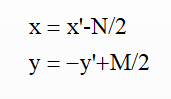逆变换为：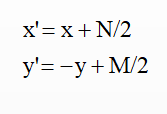于是，根据前面说的3个步骤（3次变换），旋转(顺时针旋转)的变换形式就为，3次变换就有3个矩阵：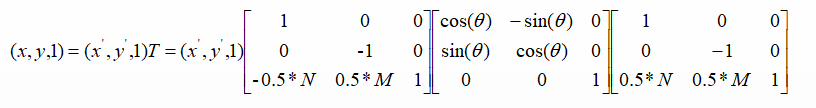反向映射
看第3个问题，在冈萨雷斯的《数字图像处理_第三版》中说的很清楚，前向映射就是根据原图用变换公式直接算出输出图像相应像素的空间位置，那么这会导致一个问题：可能会有多个像素坐标映射到输出图像的同一位置，也可能输出图像的某些位置完全没有相应的输入图像像素与它匹配，也就是没有被映射到，造成有规律的空洞（黑色的蜂窝状）。更好的一种方式是采用 反向映射（Inverse Mapping）：扫描输出图像的位置(x,y)，通过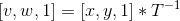（为T的逆矩阵）计算输入图像对应的位置 (v,w)，通过插值方法决定输出图像该位置的灰度值。
插值
第4个问题，采用反向映射后，需通过插值方法决定输出图像该位置的值，因此需要选择插值算法。通常有最近邻插值、双线性插值，双三次插值等，OpencV默认采用双线性插值，我们也就采用双线性插值。
2.5 基于OpenCV的实现

工具：OpenCV3.1.0+VS2013
平台：WIN10

函数原型（c++）
OpenCV仿射变换相关的函数一般涉及到warpAffine和getRotationMatrix2D这两个：

使用OpenCV函数warpAffine 来实现一些简单的重映射.
OpenCV函数getRotationMatrix2D 来获得旋转矩阵。

1、warpAffined函数详解
void boxFilter( InputArray src, OutputArray dst,
int ddepth,
Size ksize,
Point anchor = Point(-1,-1),
bool normalize = true,
int borderType = BORDER_DEFAULT );


第一个参数，InputArray类型的src，输入图像，即源图像，填Mat类的对象即可。
第二个参数，OutputArray类型的dst，函数调用后的运算结果存在这里，需和源图片有一样的尺寸和类型。
第三个参数，InputArray类型的M，2×3的变换矩阵。
第四个参数，Size类型的dsize，表示输出图像的尺寸。
第五个参数，int类型的flags，插值方法的标识符。此参数有默认值INTER_LINEAR(线性插值)，可选的插值方式如下：
INTER_NEAREST - 最近邻插值
INTER_LINEAR - 线性插值（默认值）
INTER_AREA - 区域插值
INTER_CUBIC –三次样条插值
INTER_LANCZOS4 -Lanczos插值
CV_WARP_FILL_OUTLIERS - 填充所有输出图像的象素。如果部分象素落在输入图像的边界外，那么它们的值设定为 fillval.
CV_WARP_INVERSE_MAP –表示M为输出图像到输入图像的反变换，即 。因此可以直接用来做象素插值。否则, warpAffine函数从M矩阵得到反变换。
第六个参数，int类型的borderMode，边界像素模式，默认值为BORDER_CONSTANT。
第七个参数，const Scalar&类型的borderValue，在恒定的边界情况下取的值，默认值为Scalar()，即0。

2、getRotationMatrix2D函数详解
C++: Mat getRotationMatrix2D(Point2f center, double angle, double scale)

参数：

第一个参数，Point2f类型的center，表示源图像的旋转中心。
第二个参数，double类型的angle，旋转角度。角度为正值表示向逆时针旋转（坐标原点是左上角）。
第三个参数，double类型的scale，缩放系数。## 2.6 总结

实现示例（c++)
1、旋转
	cv::Mat src = cv::imread("lenna.jpg");
cv::Mat dst;

//旋转角度
double angle = 45;

cv::Size src_sz = src.size();
cv::Size dst_sz(src_sz.height, src_sz.width);
int len = std::max(src.cols, src.rows);

//指定旋转中心（图像中点）
cv::Point2f center(len / 2., len / 2.);

//获取旋转矩阵（2x3矩阵）
cv::Mat rot_mat = cv::getRotationMatrix2D(center, angle, 1.0);

//根据旋转矩阵进行仿射变换
cv::warpAffine(src, dst, rot_mat, dst_sz);

//显示旋转效果
cv::imshow("image", src);
cv::imshow("result", dst);

cv::waitKey(0);

return 0;

2、平移
	cv::Mat src = cv::imread("lenna.jpg");
cv::Mat dst;

cv::Size dst_sz = src.size();

//定义平移矩阵
cv::Mat t_mat =cv::Mat::zeros(2, 3, CV_32FC1);

t_mat.at<float>(0, 0) = 1;
t_mat.at<float>(0, 2) = 20; //水平平移量
t_mat.at<float>(1, 1) = 1;
t_mat.at<float>(1, 2) = 10; //竖直平移量

//根据平移矩阵进行仿射变换
cv::warpAffine(src, dst, t_mat, dst_sz);

//显示平移效果
cv::imshow("image", src);
cv::imshow("result", dst);

cv::waitKey(0);

return 0;

进阶实现(根据原理自己实现)
1、旋转
/*图像旋转（以图像中心为旋转中心）*/
void affine_trans_rotate(cv::Mat& src, cv::Mat& dst, double Angle){
double angle = Angle*CV_PI / 180.0;
//构造输出图像
int dst_rows = round(fabs(src.rows * cos(angle)) + fabs(src.cols * sin(angle)));//图像高度
int dst_cols = round(fabs(src.cols * cos(angle)) + fabs(src.rows * sin(angle)));//图像宽度

if (src.channels() == 1) {
dst = cv::Mat::zeros(dst_rows, dst_cols, CV_8UC1); //灰度图初始
}
else {
dst = cv::Mat::zeros(dst_rows, dst_cols, CV_8UC3); //RGB图初始
}

cv::Mat T1 = (cv::Mat_<double>(3,3) << 1.0,0.0,0.0 , 0.0,-1.0,0.0, -0.5*src.cols , 0.5*src.rows , 1.0); // 将原图像坐标映射到数学笛卡尔坐标
cv::Mat T2 = (cv::Mat_<double>(3,3) << cos(angle),-sin(angle),0.0 , sin(angle), cos(angle),0.0, 0.0,0.0,1.0); //数学笛卡尔坐标下顺时针旋转的变换矩阵
double t3 = { { 1.0, 0.0, 0.0 }, { 0.0, -1.0, 0.0 }, { 0.5*dst.cols, 0.5*dst.rows ,1.0} }; // 将数学笛卡尔坐标映射到旋转后的图像坐标
cv::Mat T3 = cv::Mat(3.0,3.0,CV_64FC1,t3);
cv::Mat T = T1*T2*T3;
cv::Mat T_inv = T.inv(); // 求逆矩阵

for (double i = 0.0; i < dst.rows; i++){
for (double j = 0.0; j < dst.cols; j++){
cv::Mat dst_coordinate = (cv::Mat_<double>(1, 3) << j, i, 1.0);
cv::Mat src_coordinate = dst_coordinate * T_inv;
double v = src_coordinate.at<double>(0, 0); // 原图像的横坐标，列，宽
double w = src_coordinate.at<double>(0, 1); // 原图像的纵坐标，行，高
//	std::cout << v << std::endl;

/*双线性插值*/
// 判断是否越界
if (int(Angle) % 90 == 0) {
if (v < 0) v = 0; if (v > src.cols - 1) v = src.cols - 1;
if (w < 0) w = 0; if (w > src.rows - 1) w = src.rows - 1; //必须要加上，否则会出现边界问题
}

if (v >= 0 && w >= 0 && v <= src.cols - 1 && w <= src.rows - 1){
int top = floor(w), bottom = ceil(w), left = floor(v), right = ceil(v); //与映射到原图坐标相邻的四个像素点的坐标
double pw = w - top ; //pw为坐标 行 的小数部分(坐标偏差)
double pv = v - left; //pv为坐标 列 的小数部分(坐标偏差)
if (src.channels() == 1){
//灰度图像
dst.at<uchar>(i, j) = (1 - pw)*(1 - pv)*src.at<uchar>(top, left) + (1 - pw)*pv*src.at<uchar>(top, right) + pw*(1 - pv)*src.at<uchar>(bottom, left) + pw*pv*src.at<uchar>(bottom, right);
}
else{
//彩色图像
dst.at<cv::Vec3b>(i, j) = (1 - pw)*(1 - pv)*src.at<cv::Vec3b>(top, left) + (1 - pw)*pv*src.at<cv::Vec3b>(top, right) + pw*(1 - pv)*src.at<cv::Vec3b>(bottom, left) + pw*pv*src.at<cv::Vec3b>(bottom, right);
dst.at<cv::Vec3b>(i, j) = (1 - pw)*(1 - pv)*src.at<cv::Vec3b>(top, left) + (1 - pw)*pv*src.at<cv::Vec3b>(top, right) + pw*(1 - pv)*src.at<cv::Vec3b>(bottom, left) + pw*pv*src.at<cv::Vec3b>(bottom, right);
dst.at<cv::Vec3b>(i, j) = (1 - pw)*(1 - pv)*src.at<cv::Vec3b>(top, left) + (1 - pw)*pv*src.at<cv::Vec3b>(top, right) + pw*(1 - pv)*src.at<cv::Vec3b>(bottom, left) + pw*pv*src.at<cv::Vec3b>(bottom, right);
}
}
}
}
}


2、平移
/*平移变换*（以图像左顶点为原点）/
/****************************************
tx: 水平平移距离 正数向右移动 负数向左移动
ty: 垂直平移距离 正数向下移动 负数向上移动
*****************************************/
void affine_trans_translation(cv::Mat& src, cv::Mat& dst, double tx, double ty){
//构造输出图像
int dst_rows = src.rows;//图像高度
int dst_cols = src.cols;//图像宽度

if (src.channels() == 1) {
dst = cv::Mat::zeros(dst_rows, dst_cols, CV_8UC1); //灰度图初始
}
else {
dst = cv::Mat::zeros(dst_rows, dst_cols, CV_8UC3); //RGB图初始
}

cv::Mat T = (cv::Mat_<double>(3, 3) << 1,0,0 , 0,1,0 , tx,ty,1); //平移变换矩阵
cv::Mat T_inv = T.inv(); // 求逆矩阵

for (int i = 0; i < dst.rows; i++){
for (int j = 0; j < dst.cols; j++){
cv::Mat dst_coordinate = (cv::Mat_<double>(1, 3) << j, i, 1);
cv::Mat src_coordinate = dst_coordinate * T_inv;
double v = src_coordinate.at<double>(0, 0); // 原图像的横坐标，列，宽
double w = src_coordinate.at<double>(0, 1); // 原图像的纵坐标，行，高

/*双线性插值*/
// 判断是否越界

if (v >= 0 && w >= 0 && v <= src.cols - 1 && w <= src.rows - 1){
int top = floor(w), bottom = ceil(w), left = floor(v), right = ceil(v); //与映射到原图坐标相邻的四个像素点的坐标
double pw = w - top; //pw为坐标 行 的小数部分(坐标偏差)
double pv = v - left; //pv为坐标 列 的小数部分(坐标偏差)
if (src.channels() == 1){
//灰度图像
dst.at<uchar>(i, j) = (1 - pw)*(1 - pv)*src.at<uchar>(top, left) + (1 - pw)*pv*src.at<uchar>(top, right) + pw*(1 - pv)*src.at<uchar>(bottom, left) + pw*pv*src.at<uchar>(bottom, right);
}
else{
//彩色图像
dst.at<cv::Vec3b>(i, j) = (1 - pw)*(1 - pv)*src.at<cv::Vec3b>(top, left) + (1 - pw)*pv*src.at<cv::Vec3b>(top, right) + pw*(1 - pv)*src.at<cv::Vec3b>(bottom, left) + pw*pv*src.at<cv::Vec3b>(bottom, right);
dst.at<cv::Vec3b>(i, j) = (1 - pw)*(1 - pv)*src.at<cv::Vec3b>(top, left) + (1 - pw)*pv*src.at<cv::Vec3b>(top, right) + pw*(1 - pv)*src.at<cv::Vec3b>(bottom, left) + pw*pv*src.at<cv::Vec3b>(bottom, right);
dst.at<cv::Vec3b>(i, j) = (1 - pw)*(1 - pv)*src.at<cv::Vec3b>(top, left) + (1 - pw)*pv*src.at<cv::Vec3b>(top, right) + pw*(1 - pv)*src.at<cv::Vec3b>(bottom, left) + pw*pv*src.at<cv::Vec3b>(bottom, right);
}
}
}
}
}


效果
1、旋转45度2、平移相关技术文档、博客、教材、项目推荐
opencv文档: https://docs.opencv.org/3.1.0/da/d54/group__imgproc__transform.html#ga0203d9ee5fcd28d40dbc4a1ea4451983
博客：https://blog.csdn.net/weixin_40647819/article/details/87912122
https://www.jianshu.com/p/18cd12e776e1
https://blog.csdn.net/whuhan2013/article/details/53814026
python版本：https://blog.csdn.net/g11d111/article/details/79978582
https://www.kancloud.cn/aollo/aolloopencv/264331                      http://www.woshicver.com/FifthSection/4_2_%E5%9B%BE%E5%83%8F%E5%87%A0%E4%BD%95%E5%8F%98%E6%8D%A2/
2.6 总结
该部分对几何变换的平移和旋转进行了介绍，读者可根据提供的资料对相关原理进行学习，然后参考示例代码自行实现。另外读者可以尝试学习并实现其他几何变换，如偏移。


展开全文• 在传统CV领域，由于某些拍摄角度的问题，我们需要对图像进行矫正处理，而几何变换正是这个处理过程的基础，因此了解和学习几何变换也是有必要的。 这次我们带着几个问题进行，以旋转为例： 1：变换的形式（公式）是...python opencv 计算机视觉 人工智能
• 在传统CV领域，由于某些拍摄角度的问题，我们需要对图像进行矫正处理，而几何变换正是这个处理过程的基础，因此了解和学习几何变换也是有必要的。 这次我们带着几个问题进行，以旋转为例： 1：变换的形式（公...计算机视觉 opencv 人工智能 cpp
• 本文主要讲解图像傅里叶变换的相关内容，在数字图像处理中，有两个经典的变换被广泛应用——傅里叶变换和霍夫变换。其中，傅里叶变换主要是将时间域上的信号转变为频率域上的信号，用来进行图像除噪、图像增强等处理...Python图像处理 傅里叶变换 OpenCV 傅里叶逆变换
• 《Visual C++数字图像处理技术详解》以数字图像处理技术为主线，全面地介绍在Visual C++环境下进行数字图像处理程序设计的方法。内容涵盖了数字图像的变换、增强、复原、重建、形态学处理、分割、匹配、压缩编码、...
• 本文主要讲述基于VC++6.0 MFC图像处理的应用知识，主要结合自己大三所学课程《数字图像处理》及课件进行讲解，主要通过MFC单文档视图实现显示BMP图像增强处理，包括图像普通平滑、高斯平滑、不同算子的图像锐化知识...图像处理 图像增强
• 该资源主要参考我的博客【数字图像处理】六.MFC空间几何变换之图像平移、镜像、旋转、缩放详解，博客地址http://blog.csdn.net/eastmount/article/details/46345299 主要讲述基于VC++6.0 MFC图像处理的应用知识，要...
• 前一篇文章讲解了图像灰度化处理及线性变换知识，结合OpenCV调用cv2.cvtColor()函数实现图像灰度操作，本篇文章主要讲解非线性变换，使用自定义方法对图像进行灰度化处理，包括对数变换和伽马变换。本文主要讲解灰度...Python图像处理 灰度非线性变换 对数变换
• Datawhale 计算机视觉基础-图像处理（上）-Task02 几何变换 2.1 简介 该部分将对基本的几何变换进行学习，几何变换的原理大多都是相似，只是变换矩阵不同，因此，我们以最常用的平移和旋转为例进行学习。在深度学习...opencv 几何变换
• 本文主要讲述基于VC++6.0 MFC图像处理的应用知识，主要结合自己大三所学课程《数字图像处理》及课件进行讲解，主要通过MFC单文档视图实现显示BMP图片空间几何变换，包括图像平移、图形旋转、图像反转倒置镜像和图像...数字图像处理
• OpenGL视点变换，模型变换，投影变换，视口变换详解 作者：luck_net | 出处：博客园 | 2012/2/22 14:46:49 | 阅读112次 OpenGL通过相机模拟、可以实现计算机图形学中最基本的三维变换，即几何变换、投影...callback buffer
• 前一篇文章讲解了图像灰度化处理的知识，结合OpenCV调用cv2.cvtColor()函数实现图像灰度操作，使用像素处理方法对图像进行灰度化处理。本文主要讲解灰度线性变换，基础性知识希望对您有所帮助。 1.图像灰度上移变换...python图像处理 灰度处理 线性变换 opencv 图像处理
• 欢迎关注我的博客专栏“图像处理中的数学原理详解” 全文目录请见 图像处理中的数学原理详解（总纲） http://blog.csdn.net/baimafujinji/article/details/48467225     有段时间没继续更新我的“图像处理中...希尔伯特空间 图像处理 泛函分析
• 本文来源于Datawhale组队学习的教材手册，供大家参考阅读。 ...计算机视觉计算机视觉 opencvPython 图像处理 基础知识